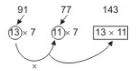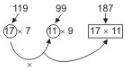Courses

# JIPMER Aptitude Mock Test 7

## 10 Questions MCQ Test JIPMER 2020: Subject wise and Full Length Mock Tests | JIPMER Aptitude Mock Test 7

Description
This mock test of JIPMER Aptitude Mock Test 7 for NEET helps you for every NEET entrance exam. This contains 10 Multiple Choice Questions for NEET JIPMER Aptitude Mock Test 7 (mcq) to study with solutions a complete question bank. The solved questions answers in this JIPMER Aptitude Mock Test 7 quiz give you a good mix of easy questions and tough questions. NEET students definitely take this JIPMER Aptitude Mock Test 7 exercise for a better result in the exam. You can find other JIPMER Aptitude Mock Test 7 extra questions, long questions & short questions for NEET on EduRev as well by searching above.
QUESTION: 1

### A boy rode his bicycle northwards, then turned left and rode one km and again turned left and rode 2 km. He found himself exactly one km west of his starting point. How far did he ride northwards initially ?

Solution:

The boy rode 2 km Northward

QUESTION: 2

### Pteridophytes are often called

Solution: Answer is b because pteridophytes spread( creep) on ground like snakes so they are called as botanical snakes .
QUESTION: 3

### Below is given statement followed by three conclusions numbered I, II and III. You have to consider the statement and the following conclusions and decide which of the conclusions is follows in the statement : Statements : a. All hotels are airports. b. All airports are belts. c. Some belts are hooks. Conclusions:I. Some belts are hotels. II. Some airports are hotels. III. Some hooks are belts.

Solution:
QUESTION: 4

Arrange the following in a meaningful order:
1. District
2. Village
3. State
4. Town

Solution:
QUESTION: 5

If Δ denotes =, + denotes > , - denotes >, '=' denotes ≠, x denotes > and ÷ denotes < then a + b -c denotes :-

Solution:
QUESTION: 6

Deepa ranks eighteenth in a class of 49 students. What is his rank from the last?

Solution:
QUESTION: 7

In the following series, how many such odd numbers are there which are divisible by 3 or 5, then followed by odd numbers and then also followed by even numbers?
12, 19, 21, 3, 25, 18, 35, 20, 22, 21, 45, 46, 47, 48, 9, 50, 52, 54, 55, 56

Solution:
QUESTION: 8

Choose the correct alternative from the given ones that will complete the series.
2, 7, 22, 67, ___, 607

Solution:

2 + 5 X 1 = 7
7 + 5 X 3 = 22
22 + 15 X 3 = 67
⇒ 67 + 45 X3 = 202

QUESTION: 9

In each of the following questions, choose that set of numbers from the four alternative sets, that is similar to the given set.

Q. Given set : (91, 77, 143)

Solution:QUESTION: 10

Below are the statements followed by two conclusions numbered I and II. You have to consider the statements and the following conclusions and decide which of the conclusion(s) follows the statement(s).

Q. Statements :
a.All cards are boxes.
b.No box is slate.
c.Some slates are tiles.
Conclusions :
I. No state is card.
II. Some tiles are boxes.

Solution: